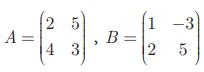Home | | Maths 10th Std | Exercise 3.18: Properties of Multiplication of Matrix

# Exercise 3.18: Properties of Multiplication of Matrix

Maths Book back answers and solution for Exercise questions - Mathematics : Properties of Multiplication of Matrix: Exercise Problem Questions with Answer

### Exercise 3.18

1. Find the order of the product matrix AB if2. If A is of order p ×q and B is of order q ×r what is the order of AB and BA?

3. has ‘a’ rows and ‘+ 3 ’ columns. B has ‘b’ rows and ‘17–b’ columns, and if both products AB and BA exist, find a, b?

4. Iffind ABBA and check if AB = BA?

5. Given thatverify that A(B+C) = AB + AC.

6. Show that the matricessatisfy commutative property AB=BA1. P × R , not defined

2. 7,10

3. 3 × 3, 4 × 2, 4 × 2, 4 × 1, 1 × 3Tags : Problem Questions with Answer, Solution | Mathematics , 10th Mathematics : UNIT 3 : Algebra
Study Material, Lecturing Notes, Assignment, Reference, Wiki description explanation, brief detail
10th Mathematics : UNIT 3 : Algebra : Exercise 3.18: Properties of Multiplication of Matrix | Problem Questions with Answer, Solution | Mathematics